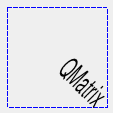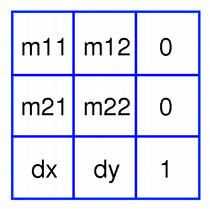#### Previous topic

QPainterPathStroker

QPainterPath

# QMatrix¶

## Detailed Description¶

The PySide.QtGui.QMatrix class specifies 2D transformations of a coordinate system.

A matrix specifies how to translate, scale, shear or rotate the coordinate system, and is typically used when rendering graphics. PySide.QtGui.QMatrix , in contrast to PySide.QtGui.QTransform , does not allow perspective transformations. PySide.QtGui.QTransform is the recommended transformation class in Qt.

A PySide.QtGui.QMatrix object can be built using the PySide.QtGui.QMatrix.setMatrix() , PySide.QtGui.QMatrix.scale() , PySide.QtGui.QMatrix.rotate() , PySide.QtGui.QMatrix.translate() and PySide.QtGui.QMatrix.shear() functions. Alternatively, it can be built by applying basic matrix operations . The matrix can also be defined when constructed, and it can be reset to the identity matrix (the default) using the PySide.QtGui.QMatrix.reset() function.

The PySide.QtGui.QMatrix class supports mapping of graphic primitives: A given point, line, polygon, region, or painter path can be mapped to the coordinate system defined by this matrix using the PySide.QtGui.QMatrix.map() function. In case of a rectangle, its coordinates can be transformed using the PySide.QtGui.QMatrix.mapRect() function. A rectangle can also be transformed into a polygon (mapped to the coordinate system defined by this matrix), using the PySide.QtGui.QMatrix.mapToPolygon() function.

PySide.QtGui.QMatrix provides the PySide.QtGui.QMatrix.isIdentity() function which returns true if the matrix is the identity matrix, and the PySide.QtGui.QMatrix.isInvertible() function which returns true if the matrix is non-singular (i.e. AB = BA = I). The PySide.QtGui.QMatrix.inverted() function returns an inverted copy of this matrix if it is invertible (otherwise it returns the identity matrix). In addition, PySide.QtGui.QMatrix provides the PySide.QtGui.QMatrix.determinant() function returning the matrix’s determinant.

Finally, the PySide.QtGui.QMatrix class supports matrix multiplication, and objects of the class can be streamed as well as compared.

### Rendering Graphics¶

When rendering graphics, the matrix defines the transformations but the actual transformation is performed by the drawing routines in PySide.QtGui.QPainter .

By default, PySide.QtGui.QPainter operates on the associated device’s own coordinate system. The standard coordinate system of a PySide.QtGui.QPaintDevice has its origin located at the top-left position. The x values increase to the right; y values increase downward. For a complete description, see the coordinate system documentation.

PySide.QtGui.QPainter has functions to translate, scale, shear and rotate the coordinate system without using a PySide.QtGui.QMatrix . For example:```def paintEvent(self, event): painter = QPainter(self) painter.setPen(QPen(Qt.blue, 1, Qt.DashLine)) painter.drawRect(0, 0, 100, 100) painter.rotate(45) painter.setFont(QFont("Helvetica", 24)) painter.setPen(QPen(Qt.black, 1)) painter.drawText(20, 10, "QMatrix") ```

Although these functions are very convenient, it can be more efficient to build a PySide.QtGui.QMatrix and call QPainter.setMatrix() if you want to perform more than a single transform operation. For example:```def paintEvent(self, event) painter = QPainter(self) painter.setPen(QPen(Qt.blue, 1, Qt.DashLine)) painter.drawRect(0, 0, 100, 100) matrix = QMatrix() matrix.translate(50, 50) matrix.rotate(45) matrix.scale(0.5, 1.0) painter.setMatrix(matrix) painter.setFont(QFont("Helvetica", 24)) painter.setPen(QPen(Qt.black, 1)) painter.drawText(20, 10, "QMatrix")```

### Basic Matrix Operations¶A PySide.QtGui.QMatrix object contains a 3 x 3 matrix. The dx and dy elements specify horizontal and vertical translation. The m11 and m22 elements specify horizontal and vertical scaling. And finally, the m21 and m12 elements specify horizontal and vertical shearing .

PySide.QtGui.QMatrix transforms a point in the plane to another point using the following formulas:

```x' = m11*x + m21*y + dx
y' = m22*y + m12*x + dy```

The point (x, y) is the original point, and (x’, y’) is the transformed point. (x’, y’) can be transformed back to (x, y) by performing the same operation on the PySide.QtGui.QMatrix.inverted() matrix.

The various matrix elements can be set when constructing the matrix, or by using the PySide.QtGui.QMatrix.setMatrix() function later on. They can also be manipulated using the PySide.QtGui.QMatrix.translate() , PySide.QtGui.QMatrix.rotate() , PySide.QtGui.QMatrix.scale() and PySide.QtGui.QMatrix.shear() convenience functions, The currently set values can be retrieved using the PySide.QtGui.QMatrix.m11() , PySide.QtGui.QMatrix.m12() , PySide.QtGui.QMatrix.m21() , PySide.QtGui.QMatrix.m22() , PySide.QtGui.QMatrix.dx() and PySide.QtGui.QMatrix.dy() functions.

Translation is the simplest transformation. Setting dx and dy will move the coordinate system dx units along the X axis and dy units along the Y axis. Scaling can be done by setting m11 and m22 . For example, setting m11 to 2 and m22 to 1.5 will double the height and increase the width by 50%. The identity matrix has m11 and m22 set to 1 (all others are set to 0) mapping a point to itself. Shearing is controlled by m12 and m21 . Setting these elements to values different from zero will twist the coordinate system. Rotation is achieved by carefully setting both the shearing factors and the scaling factors.

Here’s the combined transformations example using basic matrix operations:```def paintEvent(self, event) pi = 3.14 a = pi/180 * 45.0 sina = sin(a) cosa = cos(a) translationMatrix = QMatrix(1, 0, 0, 1, 50.0, 50.0) rotationMatrix = QMatrix(cosa, sina, -sina, cosa, 0, 0) scalingMatrix = QMatrix(0.5, 0, 0, 1.0, 0, 0) matrix = QMatrix() matrix = scalingMatrix * rotationMatrix * translationMatrix painter = QPainter(self) painter.setPen(QPen(Qt.blue, 1, Qt::DashLine)) painter.drawRect(0, 0, 100, 100) painter.setMatrix(matrix) painter.setFont(QFont("Helvetica", 24)) painter.setPen(QPen(Qt.black, 1)) painter.drawText(20, 10, "QMatrix")```

PySide.QtGui.QPainter PySide.QtGui.QTransform Coordinate System Affine Transformations Demo Transformations Example

class PySide.QtGui.QMatrix
class PySide.QtGui.QMatrix(matrix)
class PySide.QtGui.QMatrix(m11, m12, m21, m22, dx, dy)
Parameters: matrix – PySide.QtGui.QMatrix dx – PySide.QtCore.qreal dy – PySide.QtCore.qreal m21 – PySide.QtCore.qreal m11 – PySide.QtCore.qreal m22 – PySide.QtCore.qreal m12 – PySide.QtCore.qreal

Constructs an identity matrix.

All elements are set to zero except m11 and m22 (specifying the scale), which are set to 1.

Constructs a matrix that is a copy of the given matrix .

Constructs a matrix with the elements, m11 , m12 , m21 , m22 , dx and dy .

PySide.QtGui.QMatrix.__reduce__()
Return type: PyObject
PySide.QtGui.QMatrix.__repr__()
Return type: PyObject
PySide.QtGui.QMatrix.det()
Return type: PySide.QtCore.qreal

Returns the matrix’s determinant.

PySide.QtGui.QMatrix.determinant()
Return type: PySide.QtCore.qreal

Returns the matrix’s determinant.

PySide.QtGui.QMatrix.dx()
Return type: PySide.QtCore.qreal

Returns the horizontal translation factor.

PySide.QtGui.QMatrix.translate() Basic Matrix Operations

PySide.QtGui.QMatrix.dy()
Return type: PySide.QtCore.qreal

Returns the vertical translation factor.

PySide.QtGui.QMatrix.translate() Basic Matrix Operations

PySide.QtGui.QMatrix.inverted()
Return type: PyTuple

Returns an inverted copy of this matrix.

If the matrix is singular (not invertible), the returned matrix is the identity matrix. If invertible is valid (i.e. not 0), its value is set to true if the matrix is invertible, otherwise it is set to false.

PySide.QtGui.QMatrix.isIdentity()
Return type: PySide.QtCore.bool

Returns true if the matrix is the identity matrix, otherwise returns false.

PySide.QtGui.QMatrix.isInvertible()
Return type: PySide.QtCore.bool

Returns true if the matrix is invertible, otherwise returns false.

PySide.QtGui.QMatrix.m11()
Return type: PySide.QtCore.qreal

Returns the horizontal scaling factor.

PySide.QtGui.QMatrix.scale() Basic Matrix Operations

PySide.QtGui.QMatrix.m12()
Return type: PySide.QtCore.qreal

Returns the vertical shearing factor.

PySide.QtGui.QMatrix.shear() Basic Matrix Operations

PySide.QtGui.QMatrix.m21()
Return type: PySide.QtCore.qreal

Returns the horizontal shearing factor.

PySide.QtGui.QMatrix.shear() Basic Matrix Operations

PySide.QtGui.QMatrix.m22()
Return type: PySide.QtCore.qreal

Returns the vertical scaling factor.

PySide.QtGui.QMatrix.scale() Basic Matrix Operations

PySide.QtGui.QMatrix.map(a)
Parameters: a – PySide.QtGui.QPolygonF PySide.QtGui.QPolygonF

Creates and returns a PySide.QtGui.QPolygonF object that is a copy of the given polygon , mapped into the coordinate system defined by this matrix.

PySide.QtGui.QMatrix.map(r)
Parameters: r – PySide.QtGui.QRegion PySide.QtGui.QRegion

Creates and returns a PySide.QtGui.QRegion object that is a copy of the given region , mapped into the coordinate system defined by this matrix.

Calling this method can be rather expensive if rotations or shearing are used.

PySide.QtGui.QMatrix.map(x, y)
Parameters: x – PySide.QtCore.qreal y – PySide.QtCore.qreal

Maps the given coordinates x and y into the coordinate system defined by this matrix. The resulting values are put in *``tx`` and *``ty`` , respectively.

The coordinates are transformed using the following formulas:

```x' = m11*x + m21*y + dx
y' = m22*y + m12*x + dy```

The point (x, y) is the original point, and (x’, y’) is the transformed point.

Basic Matrix Operations

PySide.QtGui.QMatrix.map(a)
Parameters: a – PySide.QtGui.QPolygon PySide.QtGui.QPolygon

Creates and returns a PySide.QtGui.QPolygon object that is a copy of the given polygon , mapped into the coordinate system defined by this matrix. Note that the transformed coordinates are rounded to the nearest integer.

PySide.QtGui.QMatrix.map(x, y)
Parameters: x – PySide.QtCore.int y – PySide.QtCore.int

Maps the given coordinates x and y into the coordinate system defined by this matrix. The resulting values are put in *``tx`` and *``ty`` , respectively. Note that the transformed coordinates are rounded to the nearest integer.

PySide.QtGui.QMatrix.map(p)
Parameters: p – PySide.QtCore.QPointF PySide.QtCore.QPointF

Creates and returns a PySide.QtCore.QPointF object that is a copy of the given point , mapped into the coordinate system defined by this matrix.

PySide.QtGui.QMatrix.map(l)
Parameters: l – PySide.QtCore.QLine PySide.QtCore.QLine

Creates and returns a PySide.QtCore.QLine object that is a copy of the given line , mapped into the coordinate system defined by this matrix. Note that the transformed coordinates are rounded to the nearest integer.

PySide.QtGui.QMatrix.map(l)
Parameters: l – PySide.QtCore.QLineF PySide.QtCore.QLineF

Creates and returns a PySide.QtCore.QLineF object that is a copy of the given line , mapped into the coordinate system defined by this matrix.

PySide.QtGui.QMatrix.map(p)
Parameters: p – PySide.QtGui.QPainterPath PySide.QtGui.QPainterPath

Creates and returns a PySide.QtGui.QPainterPath object that is a copy of the given path , mapped into the coordinate system defined by this matrix.

PySide.QtGui.QMatrix.map(p)
Parameters: p – PySide.QtCore.QPoint PySide.QtCore.QPoint

Creates and returns a PySide.QtCore.QPoint object that is a copy of the given point , mapped into the coordinate system defined by this matrix. Note that the transformed coordinates are rounded to the nearest integer.

PySide.QtGui.QMatrix.mapRect(arg__1)
Parameters: arg__1 – PySide.QtCore.QRectF PySide.QtCore.QRectF

Creates and returns a PySide.QtCore.QRectF object that is a copy of the given rectangle , mapped into the coordinate system defined by this matrix.

The rectangle’s coordinates are transformed using the following formulas:

```x' = m11*x + m21*y + dx
y' = m22*y + m12*x + dy```

If rotation or shearing has been specified, this function returns the bounding rectangle. To retrieve the exact region the given rectangle maps to, use the PySide.QtGui.QMatrix.mapToPolygon() function instead.

PySide.QtGui.QMatrix.mapToPolygon() Basic Matrix Operations

PySide.QtGui.QMatrix.mapRect(arg__1)
Parameters: arg__1 – PySide.QtCore.QRect PySide.QtCore.QRect

Creates and returns a PySide.QtCore.QRect object that is a copy of the given rectangle , mapped into the coordinate system defined by this matrix. Note that the transformed coordinates are rounded to the nearest integer.

PySide.QtGui.QMatrix.mapToPolygon(r)
Parameters: r – PySide.QtCore.QRect PySide.QtGui.QPolygon

Creates and returns a PySide.QtGui.QPolygon representation of the given rectangle , mapped into the coordinate system defined by this matrix.

The rectangle’s coordinates are transformed using the following formulas:

```x' = m11*x + m21*y + dx
y' = m22*y + m12*x + dy```

Polygons and rectangles behave slightly differently when transformed (due to integer rounding), so matrix.map(QPolygon(rectangle)) is not always the same as matrix.mapToPolygon(rectangle) .

PySide.QtGui.QMatrix.mapRect() Basic Matrix Operations

PySide.QtGui.QMatrix.__ne__(arg__1)
Parameters: arg__1 – PySide.QtGui.QMatrix PySide.QtCore.bool

Returns true if this matrix is not equal to the given matrix , otherwise returns false.

PySide.QtGui.QMatrix.__mul__(o)
Parameters: o – PySide.QtGui.QMatrix PySide.QtGui.QMatrix

Returns the result of multiplying this matrix by the given matrix .

Note that matrix multiplication is not commutative, i.e. a*b != b*a.

PySide.QtGui.QMatrix.__imul__(arg__1)
Parameters: arg__1 – PySide.QtGui.QMatrix PySide.QtGui.QMatrix

Returns the result of multiplying this matrix by the given matrix .

PySide.QtGui.QMatrix.__eq__(arg__1)
Parameters: arg__1 – PySide.QtGui.QMatrix PySide.QtCore.bool

Returns true if this matrix is equal to the given matrix , otherwise returns false.

PySide.QtGui.QMatrix.reset()

Resets the matrix to an identity matrix, i.e. all elements are set to zero, except m11 and m22 (specifying the scale) which are set to 1.

PySide.QtGui.QMatrix.QMatrix() PySide.QtGui.QMatrix.isIdentity() Basic Matrix Operations

PySide.QtGui.QMatrix.rotate(a)
Parameters: a – PySide.QtCore.qreal PySide.QtGui.QMatrix

Rotates the coordinate system the given degrees counterclockwise.

Note that if you apply a PySide.QtGui.QMatrix to a point defined in widget coordinates, the direction of the rotation will be clockwise because the y-axis points downwards.

Returns a reference to the matrix.

PySide.QtGui.QMatrix.scale(sx, sy)
Parameters: sx – PySide.QtCore.qreal sy – PySide.QtCore.qreal PySide.QtGui.QMatrix

Scales the coordinate system by sx horizontally and sy vertically, and returns a reference to the matrix.

PySide.QtGui.QMatrix.setMatrix(m11, m12, m21, m22, dx, dy)
Parameters: m11 – PySide.QtCore.qreal m12 – PySide.QtCore.qreal m21 – PySide.QtCore.qreal m22 – PySide.QtCore.qreal dx – PySide.QtCore.qreal dy – PySide.QtCore.qreal

Sets the matrix elements to the specified values, m11 , m12 , m21 , m22 , dx and dy .

Note that this function replaces the previous values. PySide.QtGui.QMatrix provide the PySide.QtGui.QMatrix.translate() , PySide.QtGui.QMatrix.rotate() , PySide.QtGui.QMatrix.scale() and PySide.QtGui.QMatrix.shear() convenience functions to manipulate the various matrix elements based on the currently defined coordinate system.